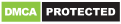# Math Solver

Genres
Version
-
Developer
-
Requires
-
Updated
-Math solver is an easy to use application for all students
it can solve step-by-step for most high school mathematic problems like:
– Finding limits
– Graphing the functions
– Polynomial equations: quaratic, cubic functions.
– Rational and irrational equations
– Solving Trigonometric equations
– Solving System fo equations: can solve linear and non-linear equations, up to 5 variables
– Solving inequality.
– Finding derivatives
– Factorization.
– Indefinite and definite integrations
– Matrix operations: addition, substraction, multiplication, inverse.
You can hit “share” to send latex code to your mail box.
This app is free
Currently available languages: Vietnamese, English
– Improve Integral

Recommended for you

You may also like